# 1.概述

• 简易平均法：包括几何平均法、算数平均法及加权平均法；
• 移动平均法：包括简单移动平均法和加权移动平均法；
• 指数平滑法：包括一次指数平滑法和二次指数平滑法，以及三次指数平滑法；
• 线性回归法：包括一元线性回归和二元线性回归。

# 2.内容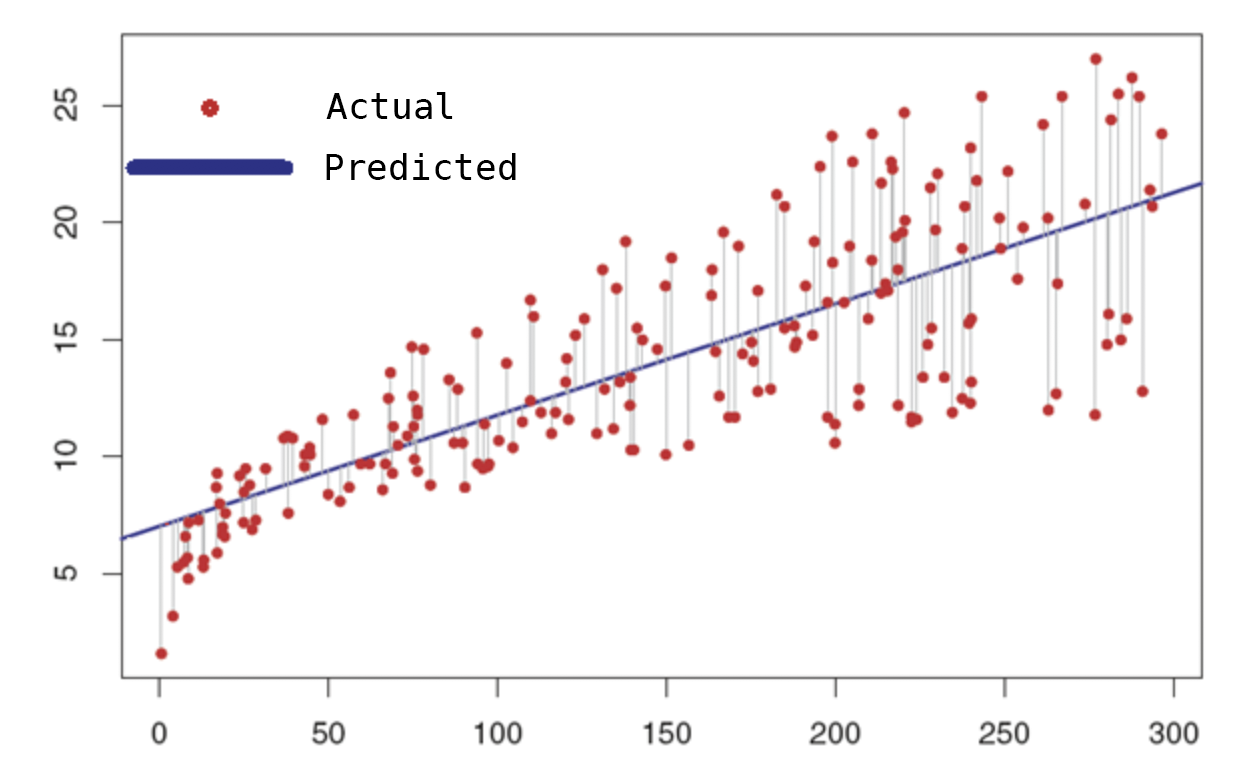## 2.1 一元线性回归

y = ax + b

y=因变量，a=系数，x=自变量，c=常数

# 读取csv文件
import pandas as pd
# 创建2D图形
import matplotlib.pyplot as plt

# 已读取csv文件

# 将名为月份的列分配给变量
Aylar = data[['Aylar']]

# 将名为Sales的列分配给变量
Satislar = data[['Satislar']]

# 使用＃sklearn库，我们导入了将数据分为测试和训练的函数
from sklearn.model_selection import train_test_split

# 我们将数据集分为测试和训练
# test_size = 0.33 数据
# x_train,y_train = 月和销售的训练集
# x_test, y_test = 月和Satislar的测试集
# test_size = 训练的数据集的2/3 1/3将保留用于测试（0.33）

x_train, x_test, y_train, y_test = train_test_split(Aylar,Satislar,test_size=0.33,random_state=0)

# 我们使用＃sklearn库包含LinearRegression类
from sklearn.linear_model import LinearRegression
# 我们从类中创建一个对象
lr = LinearRegression()

# 我们通过提供训练数据集来训练机器
lr.fit(x_train,y_train)

# 通过提供测试集，我们使Aylar可以预测Satislar
tahmin = lr.predict(x_test)

# 我们按索引号对数据进行排序，以在图表上定期显示它们
x_train = x_train.sort_index()
y_train = y_train.sort_index()

# 我们以图形形式打印屏幕
plt.plot(x_train,y_train)
plt.plot(x_test,tahmin)
plt.show()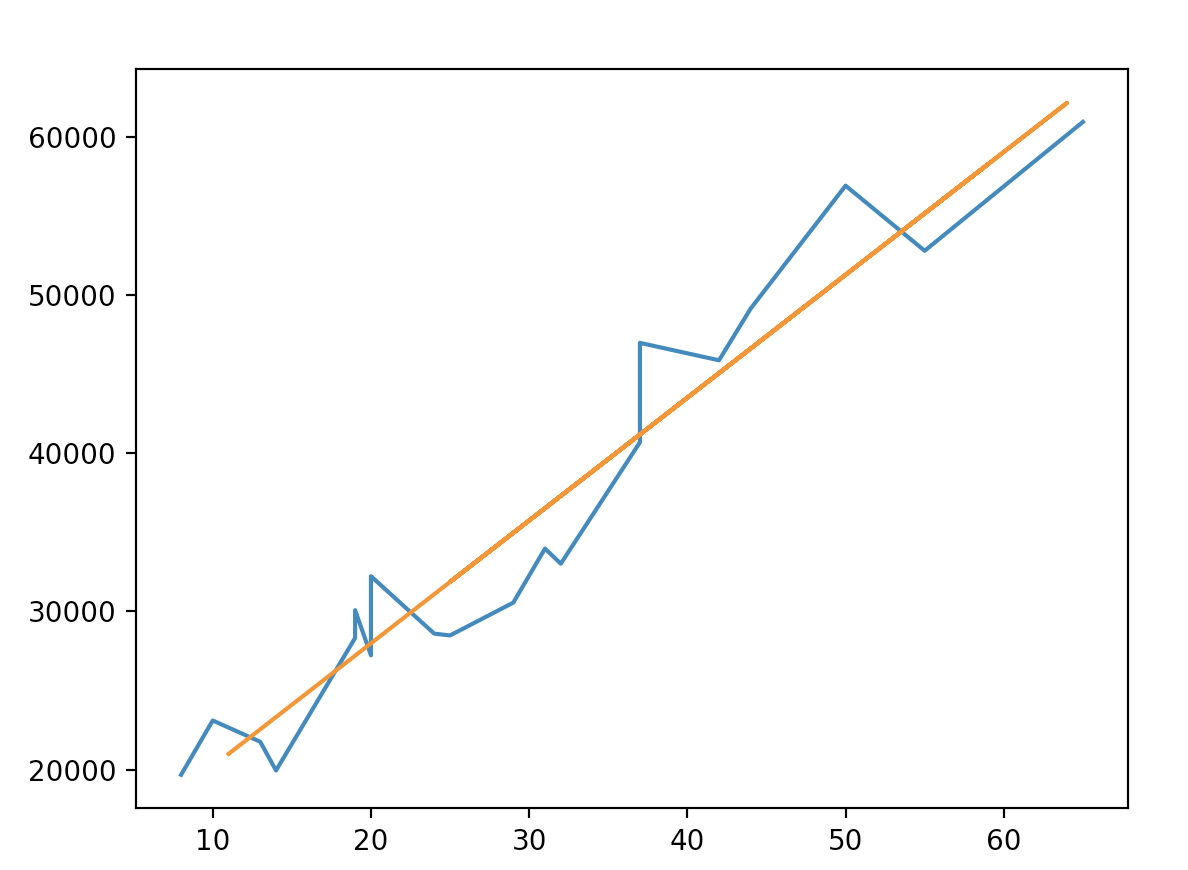## 2.2 多元线性回归

y = b0 + ax1 +bx2 + cax3 + d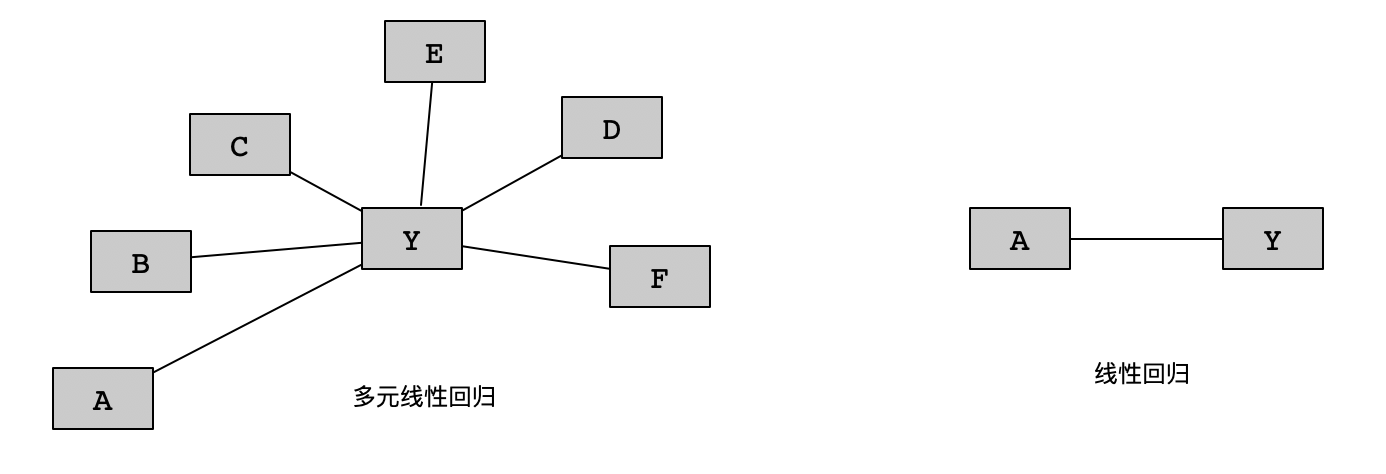### 2.2.1 虚拟变量

 年龄 身高 体重 性别 20 175 82 m 35 182 65 f 45 168 73 f 32 176 42 m

 m f 1 0 0 1 0 1 1 0

 年龄 身高 体重 性别 m f 20 175 82 m 1 0 35 182 65 f 0 1 45 168 73 f 0 1 32 176 42 m 1 0

### 2.2.2 P值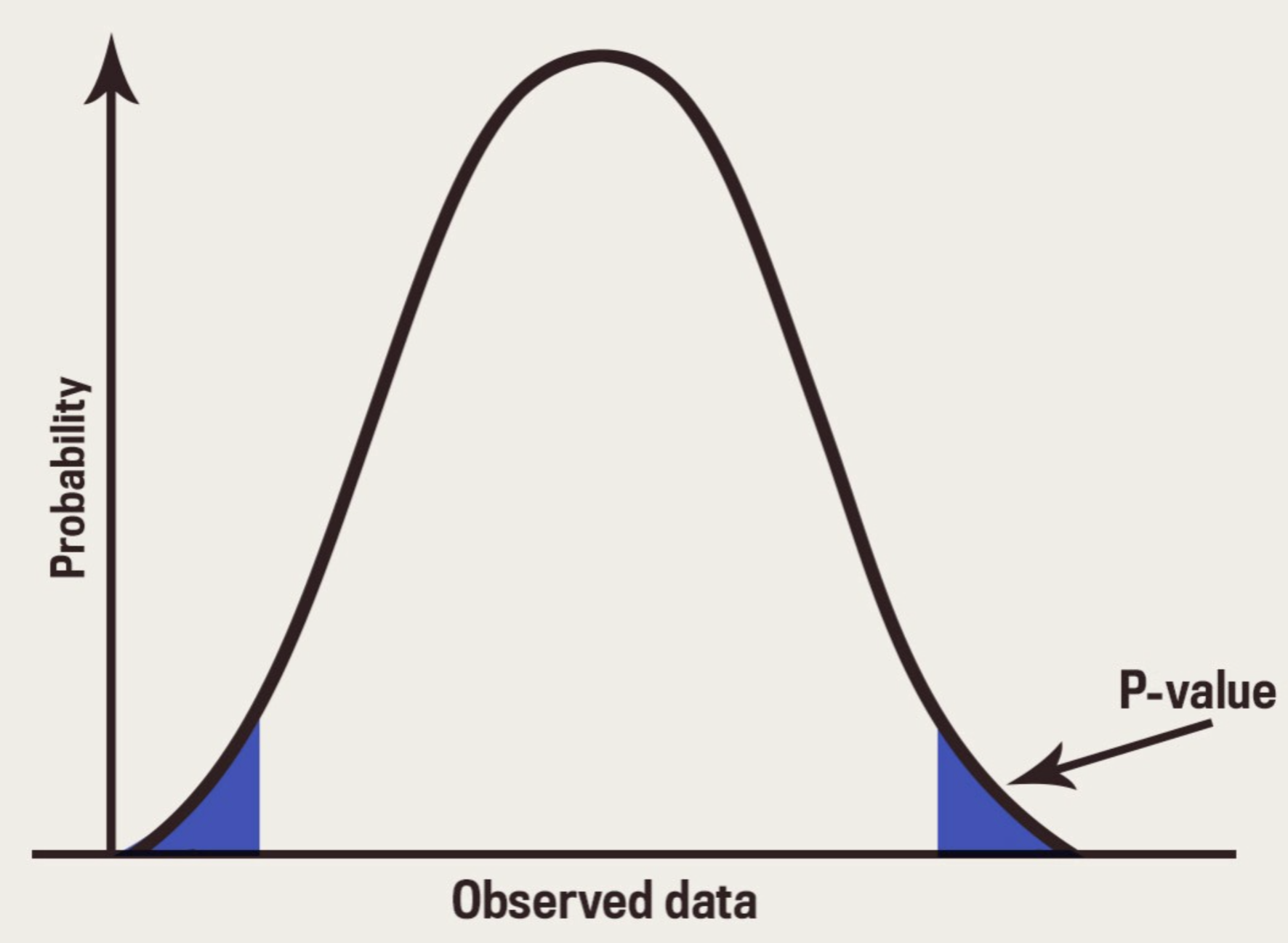P值即概率，反应某一时间发生的可能性大小。统计学根据显著性检验方法所得到的P值，一般以P小于0.05为显著，P小于0.01为非常显著，其含义是样本间的差异由抽样误差所致的概率小于0.05或者0.01。在线性回归中，P小于0.01（或者0.05）表示两个变量非常显著线性相关。

# 读取csv文件
import pandas as pd

# 已读取csv文件数据

# 要应用多元线性回归，我们将列分配给变量，以将分类数据转换为数值
cinsiyet = data.iloc[:,-1:].values

# 要应用多元线性回归，我们将列分配给变量，以将分类数据转换为数值
ulkeler = data.iloc[:,0:1].values

# 我们将要猜测的列的值分配给一个变量
boy = data.iloc[:,1:2].values

# 导入LabelEncoder类
from sklearn.preprocessing import LabelEncoder

# 我们从LabelEncoder类派生了一个对象
lb = LabelEncoder()

# 我们执行了编码过程。现在，性别和国家/地区列为数字值
cinsiyet[:,0] = lb.fit_transform(cinsiyet[:,0])
ulkeler[:,0] = lb.fit_transform(ulkeler[:,0])

# 我们导入了OneHotEncoder类
from sklearn.preprocessing import OneHotEncoder

# 我们从＃OneHotEncoder类派生了一个对象
ohe = OneHotEncoder()

# 使用＃OneHotEncoder，我们将数字列分为1和0组成的列
cinsiyet = ohe.fit_transform(cinsiyet.reshape(-1,1)).toarray()
ulkeler = ohe.fit_transform(ulkeler.reshape(-1,1)).toarray()

# 我们创建了DataFrame来重组数据
dfCinsiyet = pd.DataFrame(data=cinsiyet[:,:1],index=range(len(cinsiyet)),columns=['cinsiyet',])
dfUlkeler = pd.DataFrame(data=ulkeler,index=range(len(ulkeler)),columns=['fr','tr','us'])

# 我们合并了数据
preData = pd.concat([dfUlkeler,data.iloc[:,2:4],dfCinsiyet],axis=1)

# 我们将数据集分为测试和训练
# x_train,y_train = 训练集
# x_test, y_test = 测试集
# test_size = 训练的数据集的2/3 1/3将保留用于测试（0.33）
from sklearn.model_selection import train_test_split
x_train, x_test, y_train, y_test = train_test_split(preData,boy,test_size=0.33,random_state=0)

# 我们使用＃sklearn库包含LinearRegression类
from sklearn.linear_model import LinearRegression

# 我们从＃LinearRegression类创建一个对象
lr = LinearRegression()

# 我们通过提供训练数据集来训练机器
lr.fit(x_train,y_train)

# 通过提供＃测试集，我们使我们训练的机器能够生成估计值
result = lr.predict(x_test)
print(result)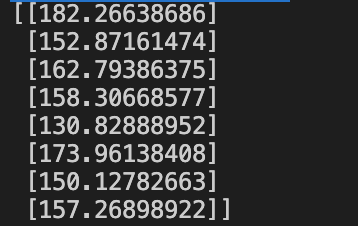### 2.2.3 向后淘汰

• 选择P值（通常此值为0.05）
• 建立一个包含所有参数的模型
• 检查每个自变量的P值，如果P值大于为模型指定的值，则将从模型中删除此自变量，然后再次运行
• 如果所有P值都小于我们确定的值，那么我们的模型基本算准备就绪了

# 读取csv文件
import pandas as pd

# 已读取csv文件数据

# 要应用多元线性回归，我们将列分配给变量，以将分类数据转换为数值
cinsiyet = data.iloc[:,-1:].values

# 要应用多元线性回归，我们将列分配给变量，以将分类数据转换为数值
ulkeler = data.iloc[:,0:1].values

# 我们将要猜测的列的值分配给一个变量
boy = data.iloc[:,1:2].values

# 导入LabelEncoder类
from sklearn.preprocessing import LabelEncoder

# 我们从LabelEncoder类派生了一个对象
lb = LabelEncoder()

# 我们执行了编码过程。现在，性别和国家/地区列为数字值
cinsiyet[:,0] = lb.fit_transform(cinsiyet[:,0])
ulkeler[:,0] = lb.fit_transform(ulkeler[:,0])

# 我们导入了OneHotEncoder类
from sklearn.preprocessing import OneHotEncoder

# 我们从＃OneHotEncoder类派生了一个对象
ohe = OneHotEncoder()

# 使用＃OneHotEncoder，我们将数字列分为1和0组成的列
cinsiyet = ohe.fit_transform(cinsiyet.reshape(-1,1)).toarray()
ulkeler = ohe.fit_transform(ulkeler.reshape(-1,1)).toarray()

# 我们创建了DataFrame来重组数据
dfCinsiyet = pd.DataFrame(data=cinsiyet[:,:1],index=range(len(cinsiyet)),columns=['cinsiyet',])
dfUlkeler = pd.DataFrame(data=ulkeler,index=range(len(ulkeler)),columns=['fr','tr','us'])

# 我们合并了数据
preData = pd.concat([dfUlkeler,data.iloc[:,2:4],dfCinsiyet],axis=1)

# 我们将数据集分为测试和训练
# x_train,y_train = 训练集
# x_test, y_test = 测试集
# test_size = 训练的数据集的2/3 1/3将保留用于测试（0.33）
from sklearn.model_selection import train_test_split
x_train, x_test, y_train, y_test = train_test_split(preData,boy,test_size=0.33,random_state=0)

# 我们使用＃sklearn库包含LinearRegression类
from sklearn.linear_model import LinearRegression

# 我们从＃LinearRegression类创建一个对象
lr = LinearRegression()

# 我们通过提供训练数据集来训练机器
lr.fit(x_train,y_train)

# 通过提供＃测试集，我们使我们训练的机器能够生成估计值
result = lr.predict(x_test)
print(result)

# 我们导入了必要的库
import statsmodels.api as sm
import numpy as np

# 我们在预处理数据中创建了一个列，并将1分配给所有值
X = np.append(arr=np.ones((len(preData),1)).astype(int),values=preData,axis=1)

# 我们处理了每一列。通过玩这部分，我们可以开发出最佳模型
X_l = preData.iloc[:,[0,1,2,3,4,5]].values

# endog = 预测的部分
# exog = 因变量
r = sm.OLS(endog=boy,exog=X_l).fit()

# 输出
print(r.summary())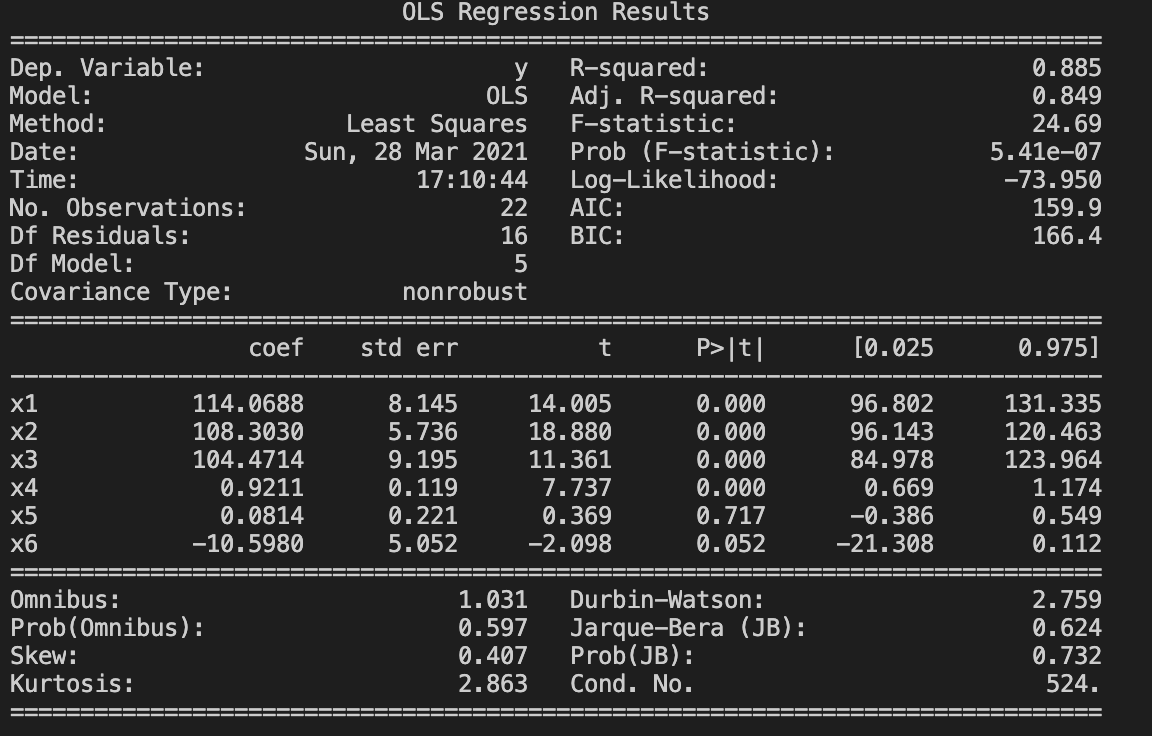在图中，P > | t | 这一列是我们的P值，在x5对应的列高于我们确定的P值（0.05），接下来，将删除此列，然后再次运行该程序，这个过程一直持续到 P > | t | 这列中所有值都小于P值为止。

## 2.3 非线性下的多项式回归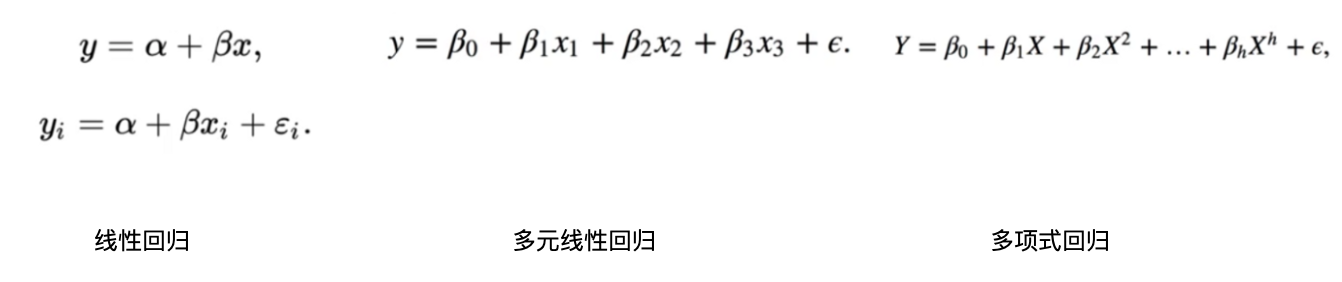在上图公式中，h是多项式的次数。它被称为h的二次方、三次方、四次方。

# 读取csv文件
import pandas as pd
# 绘制图形
import matplotlib.pyplot as plt

# 加载数据

# 将训练列的值分配给一个变量
egitim = data.iloc[:, 1:2].values

# 将maas列的值分配给一个变量
maas = data.iloc[:, -1:].values

# 导入了LinearRegression和PolynomialFeatures类
from sklearn.linear_model import LinearRegression
from sklearn.preprocessing import PolynomialFeatures

# 从LinearRegression类生成了一个对象
lr = LinearRegression()

# 我们从＃PolynomialFeatures类创建了一个对象
# 这里的度数参数是多项式的度数
# 如果我们给＃度部分加1，将会画一条直线
# 度增加得越多，我们得到的结果就越准确
poly = PolynomialFeatures(degree=4)

# 在训练机器之前，我们使用PolynomialFeatures转换训练列中的值
egitim_poly = poly.fit_transform(egitim)

# 开始训练
lr.fit(egitim_poly, maas)

# 在训练机器后做出预测
predict = lr.predict(egitim_poly)

# 以图形形式打印屏幕
plt.scatter(egitim, maas, color='red')
plt.plot(egitim, predict, color='blue')
plt.show()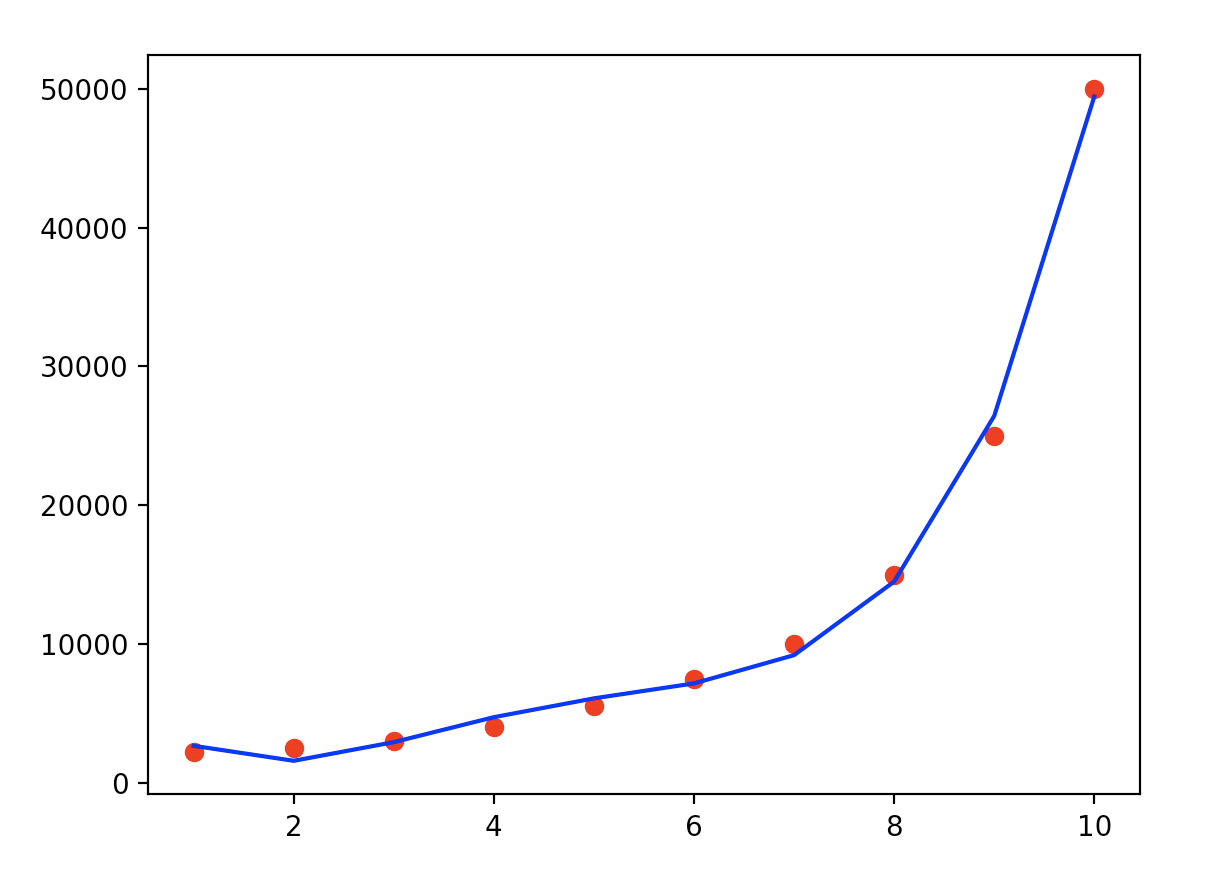更改不同的度数参数后的结果如下所示：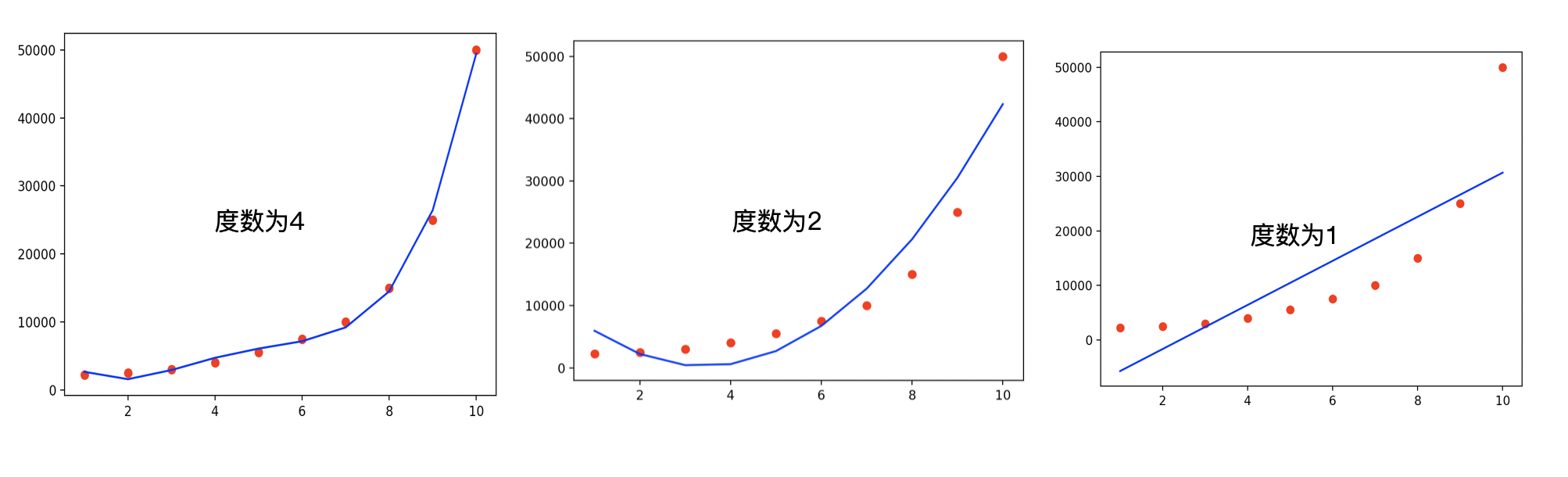# 4.结束语

QQ群（Kafka并不难学）： 825943084

### 转载请注明出处，谢谢合作！

posted @ 2021-03-28 17:40  哥不是小萝莉  阅读(612)  评论(0编辑  收藏  举报